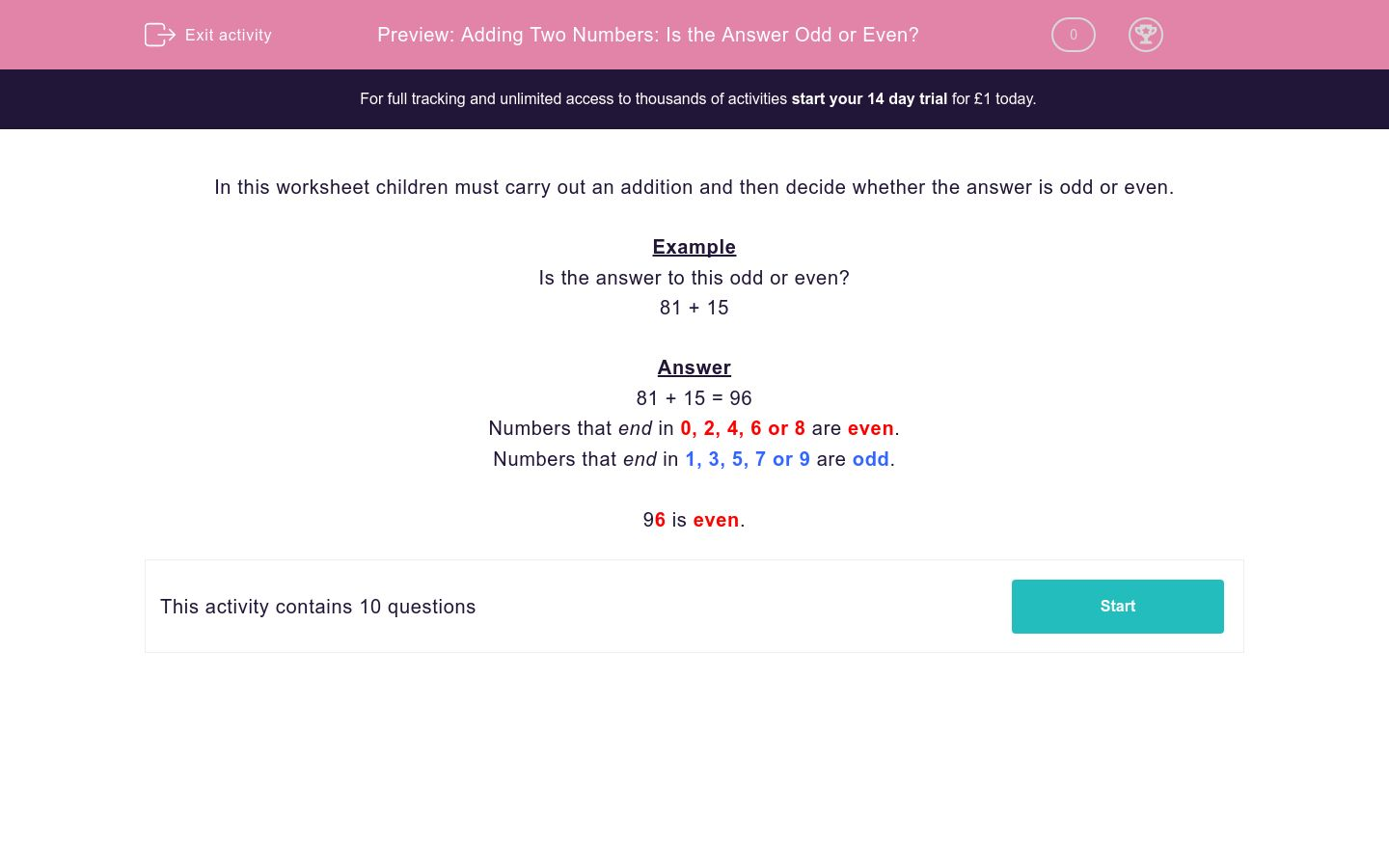### Affordable pricing from £10/month

In this worksheet, students carry out an addition of numbers with up to three digits and decide whether the sum is odd or even.Key stage:  KS 2

Curriculum topic:   Maths and Numerical Reasoning

Curriculum subtopic:   Mixed Problems

Difficulty level:### QUESTION 1 of 10

In this worksheet children must carry out an addition and then decide whether the answer is odd or even.

Example

Is the answer to this odd or even?

81 + 15

81 + 15 = 96

Numbers that end in 0, 2, 4, 6 or 8 are even.

Numbers that end in 1, 3, 5, 7 or 9 are odd.

96 is even.

Is the answer to this odd or even?

20 + 9

Even

Odd

Is the answer to this odd or even?

22 + 9

Even

Odd

Is the answer to this odd or even?

23 + 16

Even

Odd

Is the answer to this odd or even?

20 + 40

Even

Odd

Is the answer to this odd or even?

34 + 20

Even

Odd

Is the answer to this odd or even?

74 + 50

Even

Odd

Is the answer to this odd or even?

25 + 29

Even

Odd

Is the answer to this odd or even?

16 + 17

Even

Odd

Is the answer to this odd or even?

14 + 43

Even

Odd

Is the answer to this odd or even?

120 + 19

Even

Odd

• Question 1

Is the answer to this odd or even?

20 + 9

Odd
EDDIE SAYS
29 is odd.
• Question 2

Is the answer to this odd or even?

22 + 9

Odd
EDDIE SAYS
31 is odd.
• Question 3

Is the answer to this odd or even?

23 + 16

Odd
EDDIE SAYS
39 is odd.
• Question 4

Is the answer to this odd or even?

20 + 40

Even
EDDIE SAYS
60 is even.
• Question 5

Is the answer to this odd or even?

34 + 20

Even
EDDIE SAYS
54 is even.
• Question 6

Is the answer to this odd or even?

74 + 50

Even
EDDIE SAYS
124 is even.
• Question 7

Is the answer to this odd or even?

25 + 29

Even
EDDIE SAYS
54 is even.
• Question 8

Is the answer to this odd or even?

16 + 17

Odd
EDDIE SAYS
33 is odd.
• Question 9

Is the answer to this odd or even?

14 + 43

Odd
EDDIE SAYS
57 is odd.
• Question 10

Is the answer to this odd or even?

120 + 19

Odd
EDDIE SAYS
139 is odd.
---- OR ----

Sign up for a £1 trial so you can track and measure your child's progress on this activity.

### What is EdPlace?

We're your National Curriculum aligned online education content provider helping each child succeed in English, maths and science from year 1 to GCSE. With an EdPlace account you’ll be able to track and measure progress, helping each child achieve their best. We build confidence and attainment by personalising each child’s learning at a level that suits them.

Get started